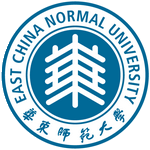王元明 教授，计算数学 华东师范大学数学系 上海市闵行区东川路500号, 200241 电话：021-54342646-215（办公室） 电子邮件：ymwang@math.ecnu.edu.cn教育工作经历 教授, 12/2003－, 华东师范大学数学系 高级访问学者, 10/2001－11/2002, 巴黎综合理工大学应用数学中心 副教授, 8/1998－11/2003, 华东师范大学数学系
 科研兴趣（研究方向） 微分方程数值解法 分数阶微分方程数值解法 主要研究方向：高阶紧有限差分方法的构造与分析
 科研项目（2010年后） 退化拟线性抛物型方程和椭圆型方程的数值分析, 上海市教委 E-研究院， E03004，01/2012－12/2015 拟线性边值问题的高精度紧有限差分方法，上海市自然科学基金，10ZR1409300，04/2010－03/2012
 学术论文（2010年后） Y.-M. Wang, T. Wang, Error analysis of a high-order compact ADI method for two-dimensional fractional convection-subdiffusion equations, Calcolo, DOI 10.1007/s10092-015-0150-3. T. Wang, Y.-M. Wang, A modified compact ADI method and its extrapolation for two-dimensional fractional subdiffusion equations, Journal of Applied Mathematics and Computing, DOI 10.1007/s12190-015-0949-8. T. Wang, Y.-M. Wang, A compact LOD method and its extrapolation for two-dimensional modified anomalous fractional sub-diffusion equations, Computers and Mathematics with Applications, 71 (2016), 147－170. C.V. Pao, Y.-M. Wang, Numerical methods for fourth-order elliptic equations with nonlocal boundary conditions, Journal of Computational and Applied Mathematics, 292 (2016), 447－468. Y.-M. Wang, Fourth-order compact finite difference methods and monotone iterative algorithms for quasi-linear elliptic boundary value problems, SIAM Journal of Numerical Analysis, 53 (2015), 1032－1057. Y.-M. Wang, A compact finite difference method for solving a class of time fractional convection-subdiffusion equations, BIT Numerical Mathematics, 55 (2015), 1187－1217. Y.-M. Wang, A compact finite difference method for a class of time fractional convection-diffusion-wave equations with variable coefficients, Numerical Algorithm, 70 (2015), 625－651. Y.-M. Wang, T. Wang, A compact locally one-dimensional method for fractional diffusion-wave equations, Journal of Applied Mathematics and Computing, 49 (2015),41－67. Y.-M. Wang, B.-Y. Guo, W.-J. Wu, Fourth-order compact finite difference methods and monotone iterative algorithms for semilinear elliptic boundary value problems, Computers and Mathematics with Applications, 68 (2014), 1671－1688. T. Wang, Y.-M. Wang, A higher-order compact LOD method and its extrapolations for nonhomogeneous parabolic differential equations, Applied Mathematics and Computation, 237 (2014), 512－530. Y.-M. Wang, Maximum norm error estimates of ADI methods for a two-dimensional fractional subdiffusion equation, Advances in Mathematical Physics, Volume 2013, Article ID 293706, 10 pages. Y.-M. Wang, W.-J. Wu, M. Scalia, Numerov's method for a class of nonlinear multipoint boundary value problems, Mathematical Problems in Engineering, Volume 2012, Article ID 316852, 29 pages. Y.-M. Wang, J. Wang, A higher-order compact ADI method with monotone iterative procedure for systems of reaction–diffusion equations, Computers and Mathematics with Applications, 62 (2011), 2434－2451. Y.-M. Wang, W.-J. Wu, Ravi P. Agarwal, A fourth-order compact finite difference method for nonlinear higher-order multi-point boundary value problems, Computers and Mathematics with Applications, 61 (2011), 3226－3245. Y.-M. Wang, A modified accelerated monotone iterative method for finite difference reaction–diffusion–convection equations, Journal of Computational and Applied Mathematics, 235 (2011), 3646－3660. Y.-M. Wang, C.-X. Liang, Ravi P. Agarwal, A block monotone iterative method for numerical solutions of nonlinear elliptic boundary value problems, Numerical Methods for Partial Differential Equations, 27 (2011), 680－701. Y.-M. Wang, On Numerov's method for a class of strongly nonlinear two-point boundary value problems, Applied Numerical Mathematics, 61 (2011), 38－52. Y.-M. Wang, Global asymptotic stability of Lotka–Volterra competition reaction–diffusion systems with time delays, Mathematical and Computer Modelling, 53 (2011), 337－346. Y.-M. Wang, Error and extrapolation of a compact LOD method for parabolic differential equations, Journal of Computational and Applied Mathematics, 235 (2011), 1367－1382. C.V. Pao, Y.-M. Wang, Nonlinear fourth-order elliptic equations with nonlocal boundary conditions, Journal of Mathematical Analysis and Applications, 372 (2010), 351－365. Y.-M. Wang, The iterative solutions of 2nth-order nonlinear multi-point boundary value problems, Applied Mathematics and Computation, 217 (2010), 2251－2259. Y.-M. Wang, On 2nth-order nonlinear multi-point boundary value problems, Mathematical and Computer Modelling, 51 (2010), 1251－1259. Y.-M. Wang, Y. Gong, Numerical solutions of a nonlinear reaction-diffusion system, International Journal of Computer Mathematics, 87 (2010), 1975－2002.
 学术团体兼职 特聘研究员，1/2003－12/2015, 上海高校计算科学 E-研究院
 教学活动 微分方程数值解法（2015－2016学年第二学期） 数值分析（2014－2015学年第二学期） 数值分析（2013－2014学年第二学期） 微分方程数值解法（2013－2014学年第一学期） 数值分析（2012－2013学年第二学期）